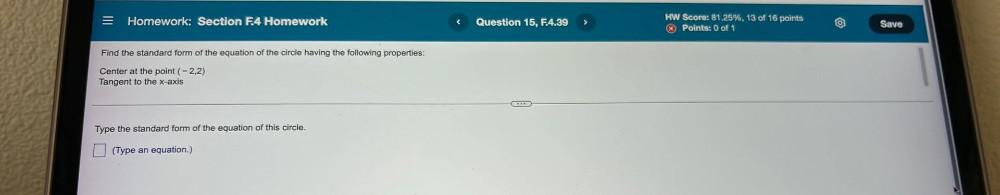Question:

# Homework: Section F.4 Homework Question 15, F.4.39 > HW Score: 81.25%, 13 of 16 points * Points: 0 of 1 Save Find the standard fHomework: Section F.4 Homework Question 15, F.4.39 > HW Score: 81.25%, 13 of 16 points * Points: 0 of 1 Save Find the standard form of the equation of the circle having the following properties: Center at the point (-2,2) Tangent to the x-axis GOOD Type the standard form of the equation of this circle. (Type an equation.)